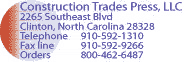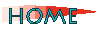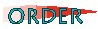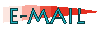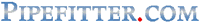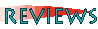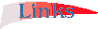Math to Build On (Hamilton)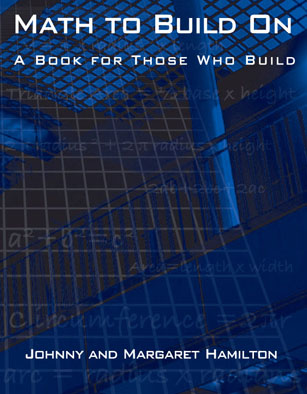A118 Dimensions (in inches) 8.5 x 11 Soft Bound 226 Pages Price \$34.95
 Table of Contents
 Acknowledgments Preface Introduction Units of Measurement Fractions and Decimals Denominators Numerators Converting Fractions to Another Term Reducing Fractions to Their Lowest Term Reducing Measuring Fractions to Their Lowest Term Increasing Fractions to Their Higher Term Converting Fractions to a Common Denominator Addition and Subtraction of Fractions Multiplication and Division of Fractions Multiplication of Fractions Division of Fractions Multiplying and Dividing Fractions by One Improper Fractions Multiplying Whole Numbers and Fractions Decimal Fractions Converting Fractions to Decimals Converting Fractions of Inches to Decimals Converting Mixed Numbers to Decimals Converting a Fraction of a Foot to a Decimal Converting Whole Inches to a Decimal of a Foot Converting Inches and a Fraction of an Inch to a Decimal of a Foot Converting Decimals to Fractions Converting a Decimal of an Inch to a Fraction Converting Inches with Decimals to Inches and Fractions Converting a Decimal of a Foot to Inches and a Fraction of an Inch Memory Aid Doubling a Measuring Fraction Halving a Measuring Fraction Halving Mixed Numbers Mixed Numbers with an Even Whole Number Mixed Numbers with an Odd Whole Number Rough Conversions Self Test Angles Angle Names 90° Angles Crossing and Parallel Lines Parallel Lines Degrees Fractions of Degrees Converting Degrees, Minutes, and Seconds to Degrees and Decimals Converting Degrees and Decimals to Degrees, Minutes, and Seconds Circles Triangles Rectangles Squares Variables and Constants Variables Constants Squaring Numbers Square Roots Right Triangles Finding the Length of the Hypotenuse Finding the Length of a Leg Names of the Sides of a Right Triangle Ratio of Sides Memory Aid Finding the Angles of a Right Triangle Using the Arc Function Keys on the Calculator Steps to Finding Angles When Two Sides Are Known Finding the Angle Using the Functions Table Complementary Angle Using the Function Keys Using the Inverse Key Using the Inverse Key with Functions Using the Inverse Key with the Arc Functions Calculating Right Triangles Using One Side and One Angle Isosceles Triangles Special Triangles The 45° Right Triangle The Equilateral Triangle The 30°-60° Right Triangle Circles PI Circumference Using the Diameter to Find the Circumference Using the Radius to Find the Circumference Arc The Arc as a Fraction of a Circle Finding Arc Length Using a Fraction of a Circle Finding the Arc Angle Using a Fraction of a Circle Concentric Arcs with the Same Angle Unit Circle Radians Finding the Arc Length Using the Radius and Radians Notes on Radians Chords Finding the Angle Measurement of a Chord Chord Length Second Module Preface Offsets Pieces of the Puzzle and Invisible Lines Simple Offsets Planes and Offset Boxes Angle of Turn Offset Triangles Simple Offset Triangles 90° Simple Offsets 45° Simple Offsets 30° Simple Offsets Odd Angle Simple Offsets Offset Turns Concentric Arcs The Take Out or Take Up of Arcs Simple Offsets with Arcs 90° Simple Offsets 30° and 45° Simple Offsets with Arcs. Odd Angle Simple Offsets with Arcs Mitered Turns Marking Miters Using the Outside Mark Back Measurement Marking Miters Using Center Mark Back Measurement Offset Turns Using Miters Odd Angle Offset Turn Mitered Offsets Area Rectangles Squares Triangles Right Triangles Isosceles Triangles Circle Side of a Cylinder Volume Rectangular Prism Calculating the Correct Amount of Concrete to Order Cubes Triangular Prism Cylinders Equal Sectors and Regular Polygons Equal Sectors Marking Equal Sectors Regular Polygons Rolling Offsets The Triangle of Roll The 45° Rolling Offset The 30° Rolling Offset The Odd Angle Rolling Offset The 90° Rolling Offset Steps for Working the Rolling Offset Notes about the Angle of Roll The Metric System Length Seeing is Believing Fractions of the Ruler Perpendicular Lines Angles Marking 60°, 30°, and 15° Angles Right Triangles Marking a 30°-60° Right Triangle Marking 45° Right Triangles Drawing Right Triangles Using Calculated Lengths Finding Degrees On Sloped Objects Isosceles Triangle Circles Arcs Concentric Arcs Cutting the 90° Elbow Into 45° Elbows. Afterword Glossary Appendix Functions Table Answers to Practices Index